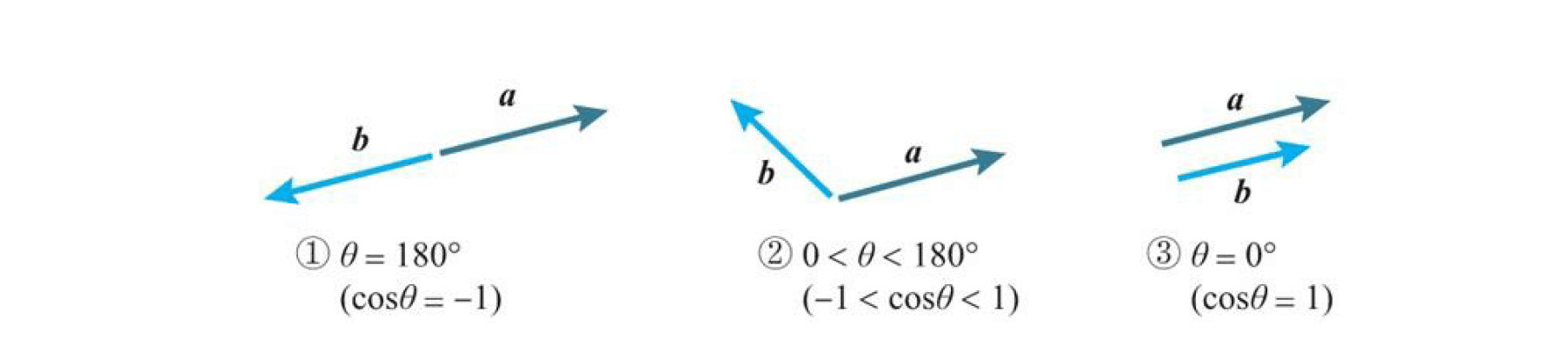# 深度学习数学基础(四)之线性代数基础

Posted by Naah on Sunday, Feb 09,2020 18:20:23

# 1 向量基础

向量就是具有大小和方向的量。

## 1.1 有向线段与向量

1. 起点$A$的位置
2. 指向$B$的方向
3. $AB$的长度，也就是大小

## 1.5 柯西·施瓦茨不等式分析发现，共有以上三种情况，在这三种情况下，我们推导出下面三种性质，后面会说到的梯度下降就是使用下面第一个性质

1. 当两个向量方向相反时，内积取得最小值。即$\cos { \theta } =-1时，\cos { \theta } \left| a \right| \left| b \right| =-\left| a \right| \left| b \right|$
2. 当两个向量不平行时，内积取平行时的中间值。即$-1<\cos { \theta } <1时，\cos { \theta } \left| a \right| \left| b \right| =\cos { \theta } \left| a \right| \left| b \right|$
3. 当两个向量方向相同时，内积取得最大值。即$\cos { \theta } =1时，\cos { \theta } \left| a \right| \left| b \right| =\left| a \right| \left| b \right|$